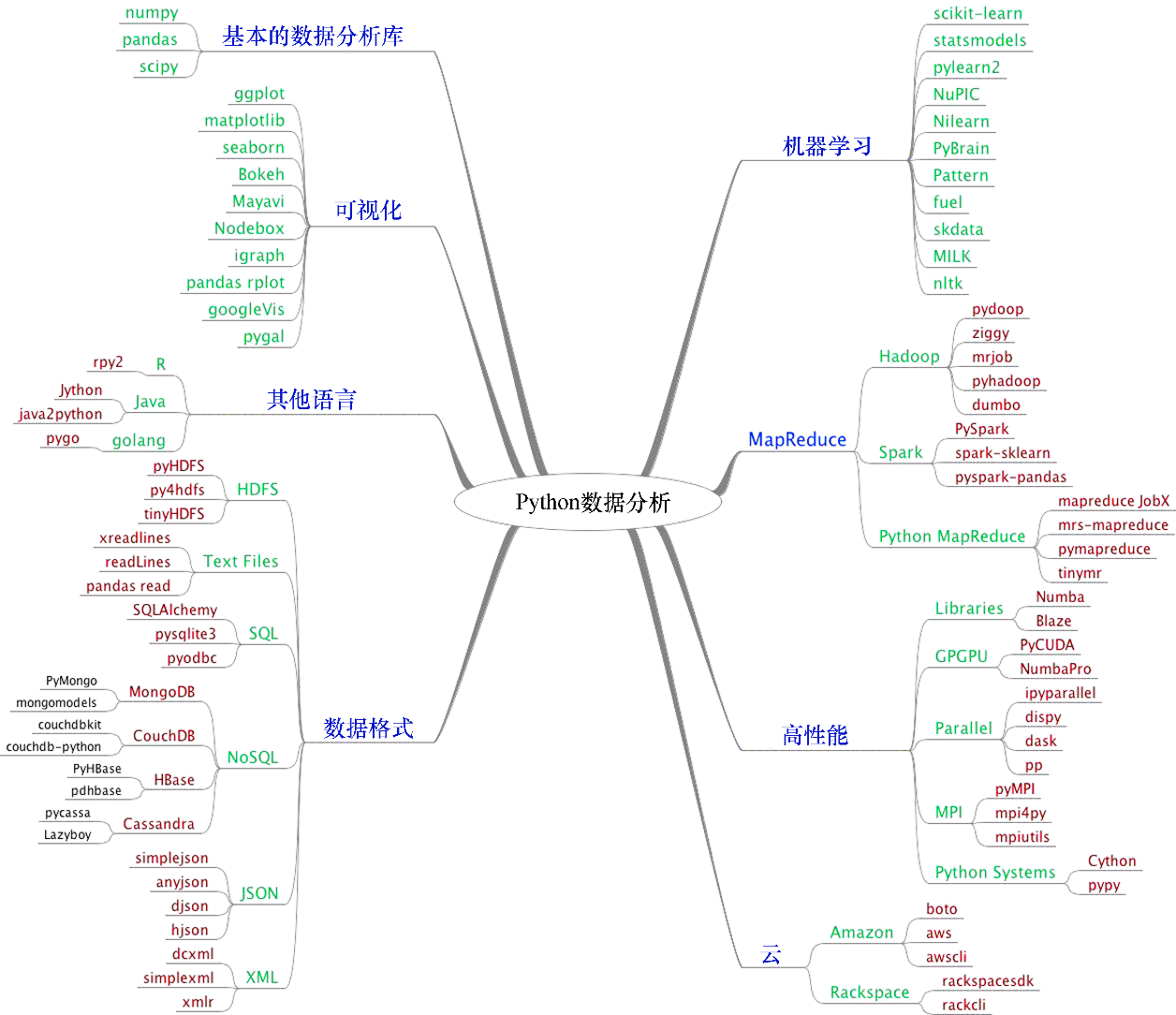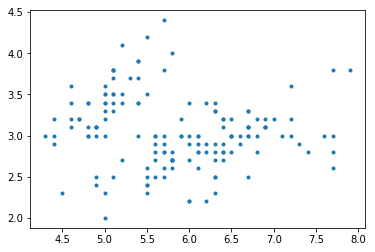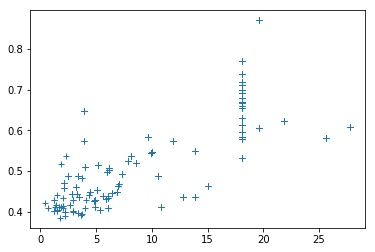# 从零开始手把手教你用Python 3做数据分析？python  同时被 2 个专栏收录8 篇文章 0 订阅

• NumPy：这是一个通用程序库，不仅支持常用的数值数组，同时提供了用于高效处理这些数组的函数。
• SciPy：这是Python的科学计算库，对NumPy的功能进行了大量扩充，同时也有部分功能是重合的。Numpy和SciPy曾经共享基础代码，后来分道扬镳了。
• Pandas：这是一个用于数据处理的程序库，不仅提供了丰富的数据结构，同时为处理数据表和时间序列提供了相应的函数。
• Matplotlib：这是一个2D绘图库，在绘制图形和图像方面提供了良好的支持。当前，Matplotlib已经并入SciPy中并支持NumPy。
• IPython：这个库为Python提供了强大的交互式Shell，也为Jupyter提供了内核，同时还支持交互式数据可视化功能。我们将在本章稍后介绍IPython shell。
• Jupyter Notebook：它提供了一个基于Web的交互式shell，可以创建和共享支持可实时代码和可视化的文档。Jupyter Notebook通过IPython提供的内核支持多个版本的Python。本章稍后将会为读者进一步介绍Jupyter Notebook。• 安装Python 3
• 将IPython用作shell
• 阅读手册页
• Jupyter Notebook
• NumPy数组
• 一个简单的应用
• 何处寻找帮助和参考资料
• 列出Python库中的模块
• 利用matplotlib可视化数据

## 1.1　安装Python 3

[外链图片转存失败,源站可能有防盗链机制,建议将图片保存下来直接上传(img-WeJA2kCm-1579577560890)(/api/storage/getbykey/original?key=1804f8abab8e0510b2a3)]　提示

## 1.2　将IPython用作shell

• Tab补全功能（Tab completion），可以帮助查找命令
• 历史记录机制
• 行内编辑
• 利用%run调用外部Python脚本
• 访问系统命令
• 访问Python的调试工具和分析工具

• 启动会话：要想使用IPython启动会话，需要在命令行中输入以下指令。

## 1.4　Jupyter Notebook

Jupyter Notebook以前被称为IPython Notebooks，它提供了一种以特殊格式创建和共享具有文本、图表和Python代码的网页的工具。

$jupyter-notebook 这时将启动notebook服务器并打开一个网页，显示该命令所在文件夹的内容。然后，你可以在Python 3中选择New | Python 3菜单项来启动一个新的notebook。 你也可以打开本书的代码包中提供的ch-01.ipynb。ch-01是一个notebook文件，其中存放了本章中简单应用程序的代码。 ## 1.5 NumPy数组 安装好NumPy后，就可以来看NumPy数组了。与Python中的列表相比，进行数值运算时NumPy数组的效率要高得多。事实上，NumPy数组是针对某些对象进行了大量的优化工作。 完成相同的运算时，与Python代码相比，NumPy代码用到的显式循环语句明显要少，因为NumPy是基于向量化的运算。还记得高等数学中标量和向量的概念吗？例如，数字2是一个标量，计算2+2时，进行的是标量加法运算。通过一组标量，我们可以构建出一个向量。用Python编程的术语来说，我们得到了一个一维数组。当然，这个概念可以扩展至更高的维度。实际上，针对两个数组的诸如加法之类的运算，可以将其转化为一组标量运算。使用纯Python时，为了完成该操作，可以使用循环语句遍历第一个数组中的每个元素，并与第二个数组中对应的元素相加。然而，在数学家眼里，这种方法过于繁琐。数学上，可以将这两个向量的加法视为单一操作。实际上，NumPy数组也可以这么做，而且它用低级C例程针对某些操作进行了优化处理，使得这些基本运算效率大为提高。关于NumPy数组将在第2章中详细介绍。 ## 1.6 一个简单的应用 假设要对向量a和b进行求和。注意，这里“向量”的含义是数学意义上的，即一个一维数组。在第4章中，将遇到一种表示矩阵的特殊NumPy数组。向量a存放的是整数0～n−1的2次幂。如果n等于3，那么a保存的是0、1或4。向量b存放的是整数0~n的3次幂，所以如果n等于3，那么向量b等于0、1或者8。如果使用普通的Python代码，该怎么做呢？ 在我们想出了一个解决方案后，可以拿来与等价的NumPy方案进行比较。 下面的函数没有借助NumPy，而是使用纯Python来解决向量加法问题。 def pythonsum(n): a = list(range(n)) b = list(range(n)) c = [] for i in range(len(a)): a[i] = i ** 2 b[i] = i ** 3 c.append(a[i] + b[i]) return c  下面是利用NumPy解决向量加法问题的函数。 def numpysum(n): a = numpy.arange(n) ** 2 b = numpy.arange(n) ** 3 c = a + b return c  注意，numpysum()无需使用for语句。此外，我们使用了来自NumPy的arange()函数，它替我们创建了一个含有整数0～n的NumPy数组。这里的arange()函数也是从NumPy导入的，所以它加上了前缀numpy。 现在到了真正有趣的地方。我们在前面讲过，NumPy在进行数组运算时，速度是相当快的。可是到底有多快呢？下面的程序代码将为我们展示numpysum()和pythonsum()这两个函数的实耗时间，这里以μs（微秒）为单位。同时，它还会显示向量sum最后面的两个元素值。下面来看使用Python和NumPy能否得到相同的答案。 #!/usr/bin/env/python import sys from datetime import datetime import numpy as np """ This program demonstrates vector addition the Python way. Run the following from the command line: python vectorsum.py n Here, n is an integer that specifies the size of the vectors. The first vector to be added contains the squares of 0 up to n. The second vector contains the cubes of 0 up to n. The program prints the last 2 elements of the sum and the elapsed time: """ def numpysum(n): a = np.arange(n) ** 2 b = np.arange(n) ** 3 c = a + b return c def pythonsum(n): a = list(range(n)) b = list(range(n)) c = [] for i in range(len(a)): a[i] = i ** 2 b[i] = i ** 3 c.append(a[i] + b[i]) return c size = int(sys.argv) start = datetime.now() c = pythonsum(size) delta = datetime.now() - start print("The last 2 elements of the sum", c[-2:]) print("PythonSum elapsed time in microseconds", delta.microseconds) start = datetime.now() c = numpysum(size) delta = datetime.now() - start print("The last 2 elements of the sum", c[-2:]) print("NumPySum elapsed time in microseconds", delta.microseconds)  对于1000个、2000个和4000个向量元素，程序的结果如下。 $ python3 vectorsum.py 1000
The last 2 elements of the sum [995007996, 998001000]
PythonSum elapsed time in microseconds 976
The last 2 elements of the sum [995007996 998001000]
NumPySum elapsed time in microseconds 87
$python3 vectorsum.py 2000 The last 2 elements of the sum [7980015996, 7992002000] PythonSum elapsed time in microseconds 1623 The last 2 elements of the sum [7980015996 7992002000] NumPySum elapsed time in microseconds 143$ python3 vectorsum.py 4000
The last 2 elements of the sum [63920031996, 63968004000]
PythonSum elapsed time in microseconds 3417
The last 2 elements of the sum [63920031996 63968004000]
NumPySum elapsed time in microseconds 237

## 1.7　从何处寻求帮助和参考资料

NumPySciPyNumPy和SciPy的主要文档网站是http://docs.scipy.org/doc/。通过该网站，您可以浏览NumPy和SciPy程序库的用户指南和参考指南，以及一些相关教程
Pandas http://pandas.pydata.org/pandas-docs/stable/
Matplotlibhttp://matplotlib.org/contents.html
IPython http://ipython.readthedocs.io/en/stable/
Jupyter Notebook　http://jupyter-notebook.readthedocs.io/en/latest/

## 1.8　查看Python库中包含的模块

ch-01.ipynb文件包含用于查看NumPy、SciPy、Pandas和Matplotlib库中的模块的代码。现在，读者不用担心这些代码的含义，只要尝试运行一下它们就行了。您可以修改其中的代码以查看其他库中的模块。

## 1.9　通过Matplotlib实现数据的可视化

\$ pip3 install scikit-learn

from sklearn.datasets import load_iris
from sklearn.datasets import load_boston

from matplotlib import pyplot as plt
%matplotlib inline

iris = load_iris()
print(iris.DESCR)
data=iris.data
plt.plot(data[:,0],data[:,1],".")boston = load_boston()
print(boston.DESCR)
data=boston.data
plt.plot(data[:,2],data[:,4],"+")## 1.10　小结11-3065
11-28377
11-25674
11-25350
11-22882
11-185456
11-16571
11-111028
11-101064
11-08190
11-042185
11-04243
11-012663
11-012449
10-28374
10-22515
10-222514
10-19697
10-193917
10-13328点击重新获取扫码支付1.余额是钱包充值的虚拟货币，按照1:1的比例进行支付金额的抵扣。
2.余额无法直接购买下载，可以购买VIP、C币套餐、付费专栏及课程。余额充值